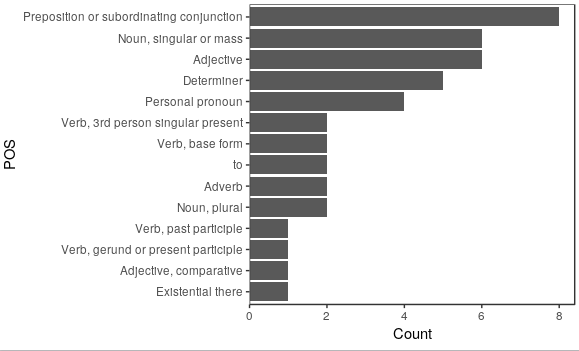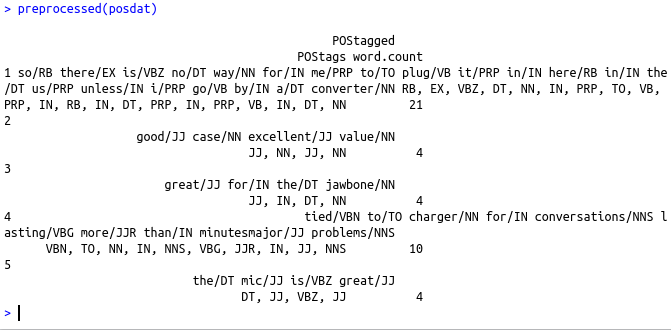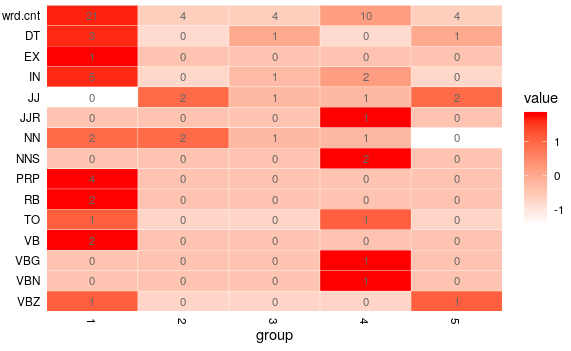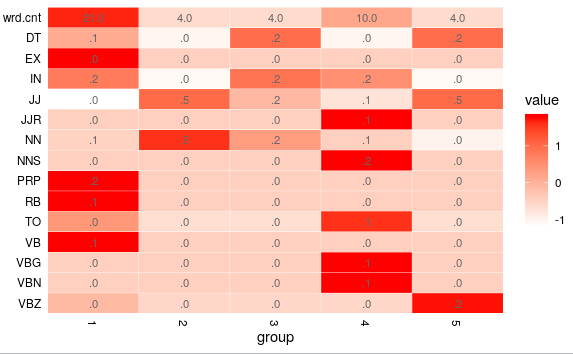• #5, First Floor, 4th Street Dr. Subbarayan Nagar Kodambakkam, Chennai-600 024 Landmark : Samiyar Madam
• pro@slogix.in
• +91- 81240 01111

How to find parts of speech in R?

Description

To find parts of speech in R

Functions Used

pos(text) – To find the parts of speech
preprocessed(posdat) – To find the words replaced with parts of speech
counts(posdat) – To find the frequency of parts of speech by row
proportions(posdat) – To find the proportion of parts of speech by row

Libraries Required :

library(qdap)

Process

Use the function pos() to find the parts of speech

Use the functions in the package to find words replaced with parts of speech,frequency of parts of speech by row,proportion of parts of speech by row

Plot the result

Sapmle Code

library(qdap)
data data1=(strsplit(data\$text,”\n”))
data2=unlist(data1[])
data3=strsplit(data2,”\t”)
data4=unlist(data3)
i=0
j=0
k=0
text=c()
pol=c()
for (i in (1:length(data4)))
{
if(i%%2!=0)
{
j=j+1
text[j]=data4[i]
}else
{
k=k+1
pol[k]=data4[i]
}
}
df=data.frame(text=text,polarity=pol,stringsAsFactors = FALSE)
posdat=pos(df\$text[1:5])
ltruncdf(posdat, 7, 4)
names(posdat)
posdat\$text #original text

#words replaced with parts of speech
preprocessed(posdat)
#frequency of parts of speech by row
counts(posdat)
#proportion of parts of speech by row
proportions(posdat)

## To plot the result
plot(preprocessed(posdat))
plot(counts(posdat))
plot(proportions(posdat))
plot(posdat)

Screenshots